Civil Engineering - Highway Engineering

Exercise :: Highway Engineering - Section 6

36.

If V is speed of a moving vehicle, r is radius of the curve, g is the acceleration due to gravity, W is the width of the carriageway, the super elevation is

 A.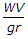B.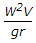C.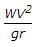D.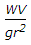E.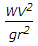Explanation:

No answer description available for this question. Let us discuss.

37.

Pick up the correct statement from the following:

 A. Various geometric design features and generally guided by ruling design speed B. The design speed for a given highway should preferably be uniform C. Abrupt change in the design speed should not be permitted D. all the above.

Explanation:

No answer description available for this question. Let us discuss.

38.

The ratio of maximum deviation angle and maximum polar deflection angle of a Lemniscate curve, is

 A. 2 B. 3 C. 4 D. 5 E. 6

Explanation:

No answer description available for this question. Let us discuss.

39.

If brakes of vehicles are effective, the vehicle-running at 30 km/hour comes to a stop in

 A. 10 metres B. 12 metres C. 15 metres D. 18 metres

Explanation:

No answer description available for this question. Let us discuss.

40.

Full amount of extra width of a pavement on the curve, is provided at

 A. beginning of the transition curve B. centre of the transition curve C. beginning of the circular curve D. centre of the circular curve.